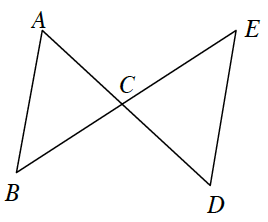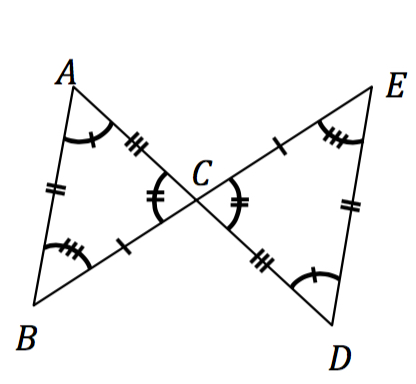### Home > CCG > Chapter 7 > Lesson 7.1.4 > Problem7-47

7-47.

If $ΔABC≅ΔDEC$, which of the statements below must be true? Justify your conclusion. Note: More than one statement may be true.

1. $\overline{AC}≅\overline{DC}$

1. $m∠B=m∠D$

1. $\overline{AB}//\overline{DE}$

1. $AD=BE$

1. None of these are true.Using the fact that the triangles are congruent, mark the angles and sides that are congruent.Determine which statements must be true. Do not rely on what simply looks true in the original diagram.